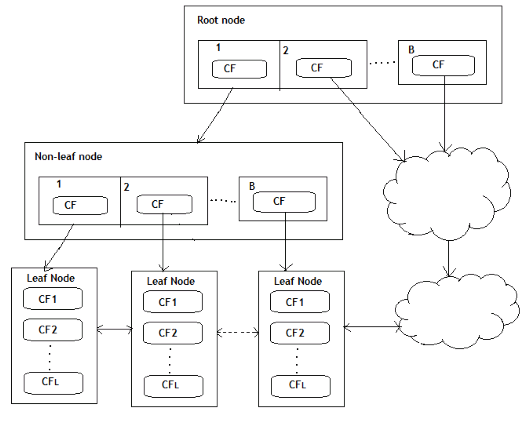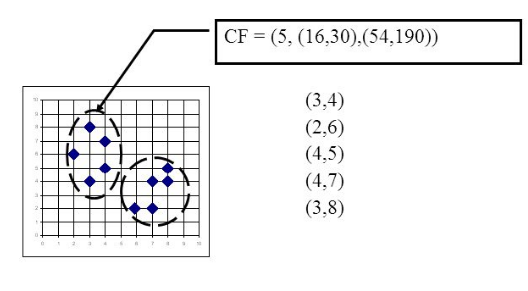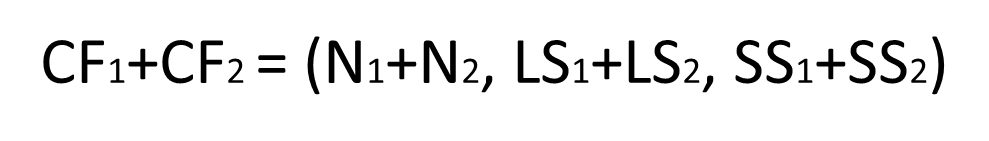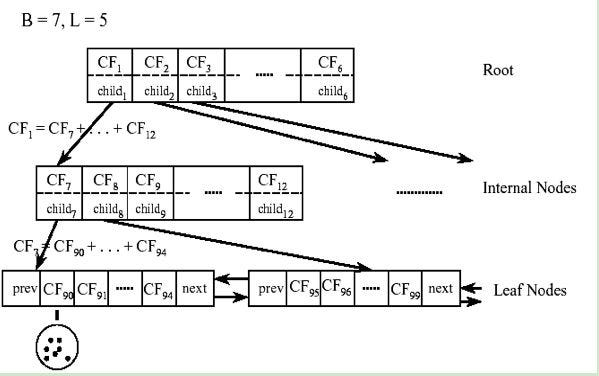1593533100

# Principle of BIRCH clustering algorithm

The BIRCH algorithm is more suitable for the case where the amount of data is large and the number of categories K is relatively large. It runs very fast, and it only needs a single pass to scan the data set for clustering. Of course, some skills are needed. Below we will summarize the BIRCH algorithm.

## BIRCH overview

BIRCH stands for Balanced Iterative Reducing and Clustering Using Hierarchies, which uses hierarchical methods to cluster and reduce data.

• BIRCH only needs to scan the data set in a single pass to perform clustering.

## How does it work?

The BIRCH algorithm uses a tree structure to create a cluster. It is generally called the Clustering Feature Tree (CF Tree). Each node of this tree is composed of several Clustering features (CF).

Clustering Feature tree structure is similar to the balanced B+ tree

From the figure below, we can see what the clustering feature tree looks like.

Each node including leaf nodes has several CFs, and the CFs of internal nodes have pointers to child nodes, and all leaf nodes are linked by a doubly linked list.From [Research Paper]

# Clustering feature (CF) and Cluster Feature Tree (CF Tree)

In the clustering feature tree, a clustering feature (CF) is defined as follows:

Each CF is a triplet, which can be represented by (N, LS, SS).

• Where N represents the number of sample points in the CF, which is easy to understand
• LS represents the vector sum of the feature dimensions of the sample points in the CF
• SS represents the square of the feature dimensions of the sample points in the CF.

For example, as shown in the following figure, in a CF of a node in the CF Tree, there are the following 5 samples (3,4), (2,6), (4,5), (4,7), ( 3,8). Then it corresponds toCF has a very good property. It satisfies the linear relationship, that is:This property is also well understood by definition. If you put this property on the CF Tree, that is to say, in the CF Tree, for each CF node in the parent node, its (N, LS, SS) triplet value is equal to the CF node pointed to The sum of the triples of all child nodes.From notes by By T, Zhang, R. Ramakrishnan

As can be seen from the above figure, the value of the triplet of CF1 of the root node can be obtained by adding the values ​​of the 6 child nodes (CF7-CF12) that it points to. In this way, we can be very efficient when updating the CF Tree.

For CF Tree, we generally have several important parameters,

• The first parameter is the maximum CF number B of each internal node,
• The second parameter is the maximum CF number L of each leaf node,
• The third parameter is for the sample points in a CF in the leaf node. It is the maximum sample radius threshold T of each CF in the leaf node. That is to say, all sample points in this CF must be in the radius In a hyper-sphere less than T.

For the CF Tree in the above figure, B = 7 and L = 5 are defined, which means that the internal node has a maximum of 7 CFs, and the leaf node has a maximum of 5 CFs.

#clustering-algorithm #machine-learning #data-science #data-mining #algorithms

## Buddha Community1600190040

## SciPy Cluster - K-Means Clustering and Hierarchical Clustering

SciPy is the most efficient open-source library in python. The main purpose is to compute mathematical and scientific problems. There are many sub-packages in SciPy which further increases its functionality. This is a very important package for data interpretation. We can segregate clusters from the data set. We can perform clustering using a single or multi-cluster. Initially, we generate the data set. Then we perform clustering on the data set. Let us learn more SciPy Clusters.

#### K-means Clustering

It is a method that can employ to determine clusters and their center. We can use this process on the raw data set. We can define a cluster when the points inside the cluster have the minimum distance when we compare it to points outside the cluster. The k-means method operates in two steps, given an initial set of k-centers,

• We define the cluster data points for the given cluster center. The points are such that they are closer to the cluster center than any other center.
• We then calculate the mean for all the data points. The mean value then becomes the new cluster center.

The process iterates until the center value becomes constant. We then fix and assign the center value. The implementation of this process is very accurate using the SciPy library.

#numpy tutorials #clustering in scipy #k-means clustering in scipy #scipy clusters #numpy1593533100

# Principle of BIRCH clustering algorithm

The BIRCH algorithm is more suitable for the case where the amount of data is large and the number of categories K is relatively large. It runs very fast, and it only needs a single pass to scan the data set for clustering. Of course, some skills are needed. Below we will summarize the BIRCH algorithm.

## BIRCH overview

BIRCH stands for Balanced Iterative Reducing and Clustering Using Hierarchies, which uses hierarchical methods to cluster and reduce data.

• BIRCH only needs to scan the data set in a single pass to perform clustering.

## How does it work?

The BIRCH algorithm uses a tree structure to create a cluster. It is generally called the Clustering Feature Tree (CF Tree). Each node of this tree is composed of several Clustering features (CF).

Clustering Feature tree structure is similar to the balanced B+ tree

From the figure below, we can see what the clustering feature tree looks like.

Each node including leaf nodes has several CFs, and the CFs of internal nodes have pointers to child nodes, and all leaf nodes are linked by a doubly linked list.From [Research Paper]

# Clustering feature (CF) and Cluster Feature Tree (CF Tree)

In the clustering feature tree, a clustering feature (CF) is defined as follows:

Each CF is a triplet, which can be represented by (N, LS, SS).

• Where N represents the number of sample points in the CF, which is easy to understand
• LS represents the vector sum of the feature dimensions of the sample points in the CF
• SS represents the square of the feature dimensions of the sample points in the CF.

For example, as shown in the following figure, in a CF of a node in the CF Tree, there are the following 5 samples (3,4), (2,6), (4,5), (4,7), ( 3,8). Then it corresponds toCF has a very good property. It satisfies the linear relationship, that is:This property is also well understood by definition. If you put this property on the CF Tree, that is to say, in the CF Tree, for each CF node in the parent node, its (N, LS, SS) triplet value is equal to the CF node pointed to The sum of the triples of all child nodes.From notes by By T, Zhang, R. Ramakrishnan

As can be seen from the above figure, the value of the triplet of CF1 of the root node can be obtained by adding the values ​​of the 6 child nodes (CF7-CF12) that it points to. In this way, we can be very efficient when updating the CF Tree.

For CF Tree, we generally have several important parameters,

• The first parameter is the maximum CF number B of each internal node,
• The second parameter is the maximum CF number L of each leaf node,
• The third parameter is for the sample points in a CF in the leaf node. It is the maximum sample radius threshold T of each CF in the leaf node. That is to say, all sample points in this CF must be in the radius In a hyper-sphere less than T.

For the CF Tree in the above figure, B = 7 and L = 5 are defined, which means that the internal node has a maximum of 7 CFs, and the leaf node has a maximum of 5 CFs.

#clustering-algorithm #machine-learning #data-science #data-mining #algorithms1617625260

## Kubernetes Cluster Federation With Admiralty

Kubernetes today is a hugely prevalent tool in 2021, and more organizations are increasingly running their applications on multiple clusters of Kubernetes. But these multiple cluster architectures often have a combination of multiple cloud providers, multiple data centers, multiple regions, and multiple zones where the applications are running. So, deploying your application or service on clusters with such diverse resources is a complicated endeavor. This challenge is what the process of a federation is intended to help overcome. The fundamental use case of a federation is to scale applications on multiple clusters with ease. The process negates the need to perform the deployment step more than once. Instead, you perform one deployment, and the application is deployed on multiple clusters as listed in the federation list.

### What Is Kubernetes Cluster Federation?

Essentially, the Kubernetes cluster federation is a mechanism to provide one way or one practice to distribute applications and services to multiple clusters. One of the most important things to note is that federation is not about cluster management, federation is about application management.

Cluster federation is a way of federating your existing clusters as one single curated cluster. So, if you are leveraging Kubernetes clusters in different zones in different countries, you can treat all of them as a single cluster.

In cluster federation, we optimize a host cluster and multiple-member clusters. The host cluster comprises all the configurations which pass on all the member clusters. Member clusters are the clusters that share the workloads. It is possible to have a host cluster also share the workload and act as a member cluster, but organizations tend to keep the host clusters separate for simplicity. On the host cluster, it’s important to install the cluster registry and the federated API. Now with the cluster registry, the host will have all the information to connect to the member Clusters. And with the federated API, you require all the controllers running on our host clusters to make sure they reconcile the federated resources. In a nutshell, the host cluster will act as a control plane and propagate and push configuration to the member clusters.

#kubernetes #cluster #cluster management #federation #federation techniques #cluster communication1623087480

## Key Data Science Algorithms Explained: From k-means to k-medoids clustering

The k-means clustering algorithm is a foundational algorithm that every data scientist should know. It is popular because it is simple, fast, and efficient. It works by dividing all the points into a preselected number (k) of clusters based on the distance between the point and the center of each cluster. The original k-means algorithm is limited because it works only in the Euclidean space and results in suboptimal cluster assignments when the real clusters are unequal in size. Despite its shortcomings, k-means remains one of the most powerful tools for clustering and has been used in healthcare, natural language processing, and physical sciences.

Extensions of the k-means algorithms include smarter starting positions for its k centers, allowing variable cluster sizes, and including more distances than Euclidean distance. In this article, we will focus on methods like PAMCLARA, and CLARANS, which incorporate distance measures beyond the Euclidean distance. These methods are yet to enjoy the fame of k-means because they are slower than k-means for large datasets without a comparable gain in optimality. However, as we will see in this article, researchers have developed newer versions of these algorithms that promise to provide better accuracy and speeds than k-means.

#### What are the shortcomings of k-means clustering?

For anyone who needs a quick reminder, StatQuest has a great video on k-means clustering.

For this article, we will focus on where k-means fails. Vanilla k-means, as explained in the video, has several disadvantages:

1. It is difficult to predict the correct number of centroids (k) to partition the data.
2. The algorithm always divides the space into k clusters, even when the partitions don’t make sense.
3. The initial positions of the k centroids can affect the results significantly.
4. It does not work well when the expected clusters differ in size and density.
5. Since it is a centroid-based approach, outliers in the data can drag the centroids to inaccurate centers.
6. Since it is a hard clustering method, clusters cannot overlap.
7. It is sensitive to the scale of the dimensions, and rescaling the data can change the results significantly.
8. It uses the Euclidean distance to divide points. The Euclidean distance becomes ineffective in high dimensional spaces since all points tend to become uniformly distant from each other. Read a great explanation here.
9. The centroid is an imaginary point in the dataset and may be meaningless.
10. Categorical variables cannot be defined by a mean and should be described by their mode.

The above figure shows an example of k-means clustering of the mouse data set using k-means, where k-means performs poorly due to varying cluster sizes.

#### Introducing Partitioning Around Medoids (PAM) algorithm

Instead of using the mean of the cluster to partition, the medoid, or the most centrally located data point in the cluster can be used to partition the data points; The medoid is the least dissimilar point to all points in the cluster. The medoid is also less sensitive to outliers in the data. These partitions can also use arbitrary distances instead of relying on the Euclidean distance. This is the crux of the clustering algorithm named Partition Around Medoids (PAM), and its extensions CLARA and CLARANS. Watch this video for a succinct explanation of the method.

In short, the following are the steps involved in the PAM method (reference):

#### Improving PAM with sampling

The time complexity of the PAM algorithm is in the order of O(k(n - k)2), which makes it much slower than the k-means algorithm. Kaufman and Rousseeuw (1990) proposed an improvement that traded optimality for speed, named CLARA (Clustering For Large Applications). In CLARA, the main dataset is split into several smaller, randomly sampled subsets of the data. The PAM algorithm is applied to each subset to obtain the medoids for each set, and the set of medoids that give the best performance on the main dataset are kept. Dudoit and Fridlyand (2003) improve the CLARA workflow by combining the medoids from different samples by voting or bagging, which aims to reduce the variability that would come from applying CLARA.

Another variation named CLARANS (Clustering Large Applications based upon RANdomized Search) (Ng and Han 2002) works by combining sampling and searching on a graph. In this graph, each node represents a set of k medoids. Each node is connected to another node if the set of k medoids in each node differs by one. The graph can be traversed until a local minimum is reached, and that minimum provides the best estimate for the medoids of the dataset.

#### Making PAM faster

Schubert and Rousseeuw (2019) proposed a faster version of PAM, which can be extended to CLARA, by changing how the algorithm caches the distance values. They summarize it well here:

“This caching was enabled by changing the nesting order of the loops in the algorithm, showing once more how much seemingly minor-looking implementation details can matter (Kriegel et al., 2017). As a second improvement, we propose to find the best swap for each medoid and execute as many as possible in each iteration, which reduces the number of iterations needed for convergence without loss of quality, as demonstrated in the experiments, and as supported by theoretical considerations. In this article, we proposed a modification of the popular PAM algorithm that typically yields an O(k) fold speedup, by clever caching of partial results in order to avoid recomputation.”

In another variation, Yue et al. (2016) proposed a MapReduce framework for speeding up the calculations of the k-medoids algorithm and named it the K-Medoids++ algorithm.

More recently, Tiwari et al. (2020) cast the problem of choosing k medoids into a multi-arm bandit problem and solved it using the Upper Confidence Bound algorithm. This variation was faster than PAM and matched its accuracy.

#2020 dec tutorials #overviews #algorithms #clustering #explained1601271540

## Clustering Techniques

Clustering falls under the unsupervised learning technique. In this technique, the data is not labelled and there is no defined dependant variable. This type of learning is usually done to identify patterns in the data and/or to group similar data.

In this post, a detailed explanation on the type of clustering techniques and a code walk-through is provided.

#k-means-clustering #hierarchical-clustering #clustering-algorithm #machine-learning